A tuplet is a group of notes evenly condensed or expanded over a specific duration of time. For instance: A group of three eighth notes may be played within the space of one quarter note. The tuplet sign is normally placed above or below the group of notes outside the musical staff.A tuplet can be any number of notes of any duration, grouped by a sign, to indicate that it should be played within a measured amount of time as it would normally be indicated by another group of note values. The following are tuplet note groupings with their technical terms.

#### Term Number of Notesduplet two triplet three quadruplet four quintuplet five sextuplet six septuplet seven octuplet eight nontuplet nine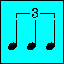A tuplet may be represented with a number in three ways. A number within a bracket helps to identify tuplet groupings that are not beamed. Such notes are anything greater or equal in value than a quarter note.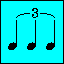A slurred tuplet identifies a group of notes to be played legato. It is much more simple to read than having bracket tuplets combined with slurs.Sometimes a tuplet grouping may be represented by a number only. This can be used with beamed notes only. Beamed notes are anything equal or smaller in value than an eighth note.

The ratio of the tuplet groupings depend very much on the time signature. The first number of the ratio indicates how many notes there are in the tuplet. The second number indicates how many note values that tuplet will occupy. Therefore, three notes in the space of two means that a triplet grouped with notes of a particular value takes up the space of two notes of the same value.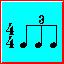In simple time, the second number of the ratio will either be a two, a four or an eight. The first number of the ratio will always be bigger than the second number providing it remains less than the second number's following value. (Select Simple Time Tuplets.)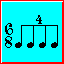In compound time, the second number of the ratio will either be a three, a six or a nine. The first number of the ratio follows the same rules as in simple time. The only exception is the 2:3 ratio where two notes fall within the space of three. (Select Compound Time Tuplets.)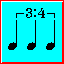There are two cases when the ratios should be considered irregular. One irregularity is when the first number of the ratio is smaller than the second. The other is when the second number does not equal to any of the standard numerical values in simple or compound time (as mentioned above). It is better to put the entire ratio in the tuplet figure to facilitate reading.